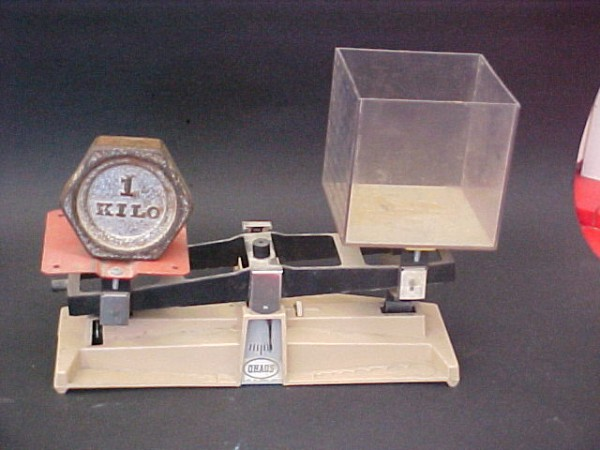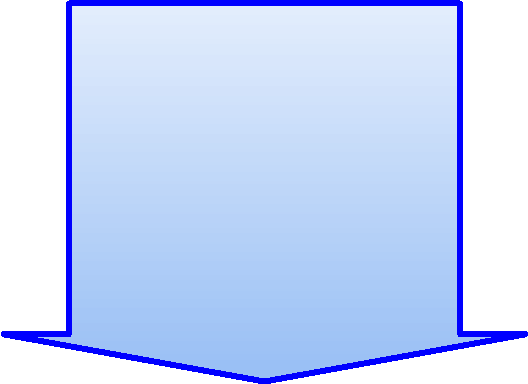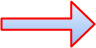But to understand the Metric System very well you must understand the cubic decimeter. Design and make one yourself. It is very important! Making a cubic decimeter will give you a much better understanding of length, area and volume. It will help you understand Canada's and the World's standard measurement system.

The Cubic Decimeter
(dm³)

The symbol (dm³) stands for a cubic decimeter.
The (dm) stands for decimeter and the small (³) tells you that its a cube.

Cube
A cube is a three-dimensional solid object. A dimension is a measurement.   Understanding the (dm³) in measurement is as important as knowing the alphabet for reading. If you want to read or write you need to memorize the alphabet. If you want to understand a big part of measurement, geometry and the metric system you need to understanding the (dm³).

dm³
The little three tells you that a metric cube measurement has length, width and debth.
dm²The little two tells you that a metric polygon measurement has length and width.

The Metric System

Plastic Cubic DecimeterFill the plastic cubic decimeter  with water, place it on a scale opposite to a kilogram and the water will weigh exactly one kilogram.
The Kilogram and The Cubic DecimeterKilogramBalance Scale

Focus on the picture of the balance scale. A balance scale is a measuring instrument. It is used to weigh the mass of something.

A balance scale is used in combination with a standard set of metric weights. When the scale is in balance it will tell you how much mass something has. The standard unit of measurement for mass is the kilogram or kg.

The balance beam has two support areas, or pans. The support areas are opposite to each other with the balance needle centered between them. When the two pans contain exactly the same mass, the beam is in balance. You will know it is in balance because the needle will be in the center.

Focus again on the balance scale. It has a kilogram weight on the left and a plastic cubic decimeter container on the right. What you cant see are the three small weights hidden behind the kilogram (kg) weight.

Before I took this picture, I placed the plastic cubic decimeter container on the right side of the balance scale. Then I experimented with weights on the left side until the scale was in balance. For this plastic container, it took three very small weights. I am not going to tell you what they are because the mass of your cubic decimeter container may be different than the one I used.

Once you have the balance scale, the plastic cubic decimeter container, a one-kilogram weight, a variety of small gram weights, a metric measuring cup, and water, (a little food colouring helps), you are ready to perform the experiment.

Once you have properly organized your tools, instruments and materials, it is time to perform the experiment.

Start by placing the plastic cubic decimeter on the balance. If you are right handed, place it on the right side. If you pour with your left hand, place it on the left side. Use some small gram weights and put the plastic container it in balance. Then place the one kilogram weight on the scale opposite the plastic container.

Fill a measuring cup with exactly one liter of water. If you can, add a little colour. Pour the water, very carefully, into the cubic decimeter container. When this experiment is completed properly, you will see the correlation between the capacity of one liter of water, the volume of one cubic decimeter and the mass of a one-kilogram weight.